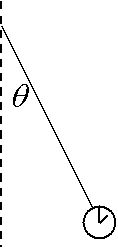# Physics Hard Forces Problem

## Homework Statement

An airplane begins its takeoff sequence moving with a constant acceleration a. A passenger holds up a pocketwatch during the takeoff sequence and notices that the watch makes an angle θ = 14° with the vertical, and that 13.3 seconds pass before the plane leaves the runway.

(a) What is the plane's constant acceleration?

(b) How far does the plane travel on the runway?

F = ma

## The Attempt at a Solution

There is no given mass, I don't know how to find it without it.

## The Attempt at a Solution

NascentOxygen
Staff Emeritus
The watch lanyard makes an angle determined by two perpendicular forces. One force is due to gravity acting on the watch's mass, m, the other due to acceleration of that same mass.

The watch lanyard makes an angle determined by two perpendicular forces. One force is due to gravity acting on the watch's mass, m, the other due to acceleration of that same mass.

Yes, but when you don't have the mass of the watch itself, how would you be able to solve it, all it gives you is the angle measure and the force of gravity, which is 10.

Here's a picture if it helps,NascentOxygen
Staff Emeritus
Yes, but when you don't have the mass of the watch itself, how would you be able to solve it,
Then you call its mass m kg, and work out the vector forces due to gravity and horizontal acceleration both in terms of m kg. What will the magnitudes of each force be?

PeterO
Homework Helper
Yes, but when you don't have the mass of the watch itself, how would you be able to solve it, all it gives you is the angle measure and the force of gravity, which is 10.

Here's a picture if it helps,Don't forget that the person across the aisle, holding their watch up like you, will see their watch hang at the same angle, and their watch has a mass of precisely 0.21 kg. Does that help?